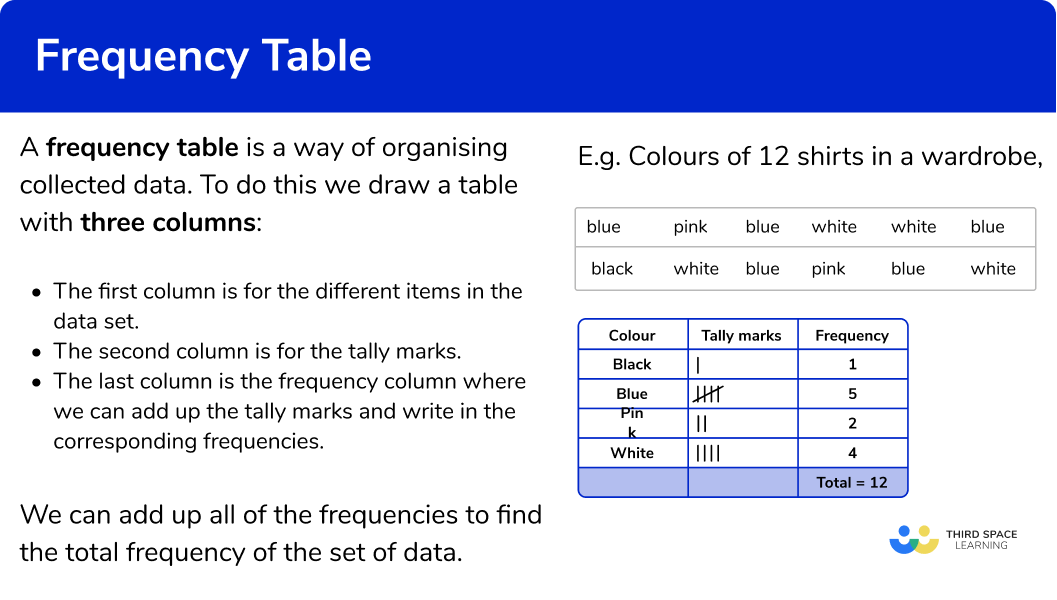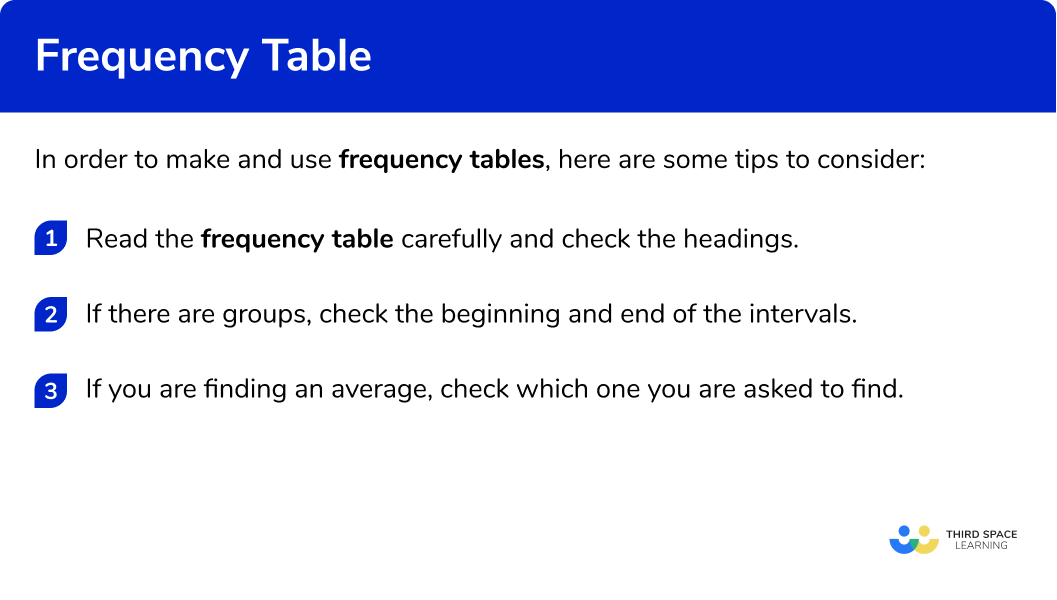GCSE Maths Statistics

Frequency Tables

# Frequency Table

Here we will learn about frequency tables, including what a frequency table is and how to make a frequency table.  We will also look at how they can be used to help analyse a set of data.

There are also frequency table worksheets based on Edexcel, AQA and OCR exam questions, along with further guidance on where to go next if you’re still stuck.

## What is a frequency table?

A frequency table is a way of organising collected data.

To do this we draw a table with three columns:

• The first column is for the different items in the data set.
• The second column is for the tally marks.
• The last column is the frequency column where we can add up the tally marks and write in the corresponding frequencies.

We can add up all of the frequencies to find the total frequency of the set of data.

For example,

Organise the colours of the 12 shirts in a wardrobe into a frequency table.

blue       pink      blue    white    white    blue

black     white     blue     pink     blue     white

The total number of shirts is 12.

### What is a frequency table?### Averages from frequency tables

We use frequency tables to find descriptive statistics.  These are values which help describe the set of data such as the mean, median and mode of a set of data.

For example,

A frequency table showing the ages of 25 students on a college course.

The mode is 18

The median is the 13^{th} value which is 18

The mean can be calculated using the total of all the values, divided by the total of the frequencies, n.

\text{mean}=\frac{\text{total}}{n}=\frac{(18\times 15)+(19\times 6)+(20\times 4)}{25}=\frac{464}{25}=18.56

Step-by-step guide: Averages from a frequency table

### Grouped frequency table

Numerical data can also be organised into grouped data.  Here the data is put into different classes with class intervals.

For example,

A grouped frequency table showing the heights of 15 students.

The mode class is 140<h\leq150

The median is the 8^{th} value which is in the 140<h\leq150 class interval

We can only calculate an estimate for the mean using the midpoints of the class intervals.  The total of the frequencies is n.

\text{mean}=\frac{\text{total}}{n}=\frac{(135\times 3)+(145\times 7)+(155\times 5)}{15}=\frac{2195}{15}=146.3 (1 d.p.)

Step-by-step guide: Grouped frequency table

Frequency tables can be used to draw bar charts, pie charts or histograms.  They can also be used to find cumulative frequency which in turn can be used to estimate median values and upper and lower quartiles for grouped data.

## How to make and use frequency tables

In order to make and use frequency tables, here are some tips to consider:

• If there are groups, check the beginning and end of the intervals.
• If you are finding an average, check which one you are asked to find.

### Explain how to make and use frequency tables## Frequency table examples

### Example 1: categorical data

Here are the makes of 20 cars.

Ford      BMW      Honda      Honda      VW     Toyota

Ford      Toyota     Honda     Toyota      Ford      Honda

Honda     VW       Toyota      Honda      Ford      Ford

• Complete the frequency table.

• Go along the data set and for each item put a tally mark in the table.  When you have finished, add up the tally marks to find the frequencies.

• Add up the frequencies in the final column to get the total number of items in the data set.

### Example 2: numerical data

Here are the temperatures at midday for 7 days (in ०C)

• Complete the frequency table.

• Go along the data set and for each item put a tally mark in the table.  When you have finished, add up the tally marks to find the frequencies.

• Add up the frequencies in the final column to get the total number of items in the data set.

### Example 3: grouped data

Here are the speeds of 20 vehicles, to the nearest mph.

• Complete the grouped frequency table:

• Go along the data set and for each item put a tally mark in the table.  When you have finished, add up the tally marks to find the frequencies.  Take care with the inequalities and the minimum value and the maximum value in each class interval.

• Add up the frequencies in the final column to get the total number of items in the data set.

### Example 4: average from a frequency table

Write down the mode.

• The mode is ‘Car’ as it has the highest frequency.  The modal vehicle is ‘Car’.

### Example 5: averages from a frequency table

Find the mode, median and mean from this frequency table

• The mode is 20^{\circ}

• The median is the 11^{th} value which is 19^{\circ}

• The mean is

\frac{(17\times 1)+(18\times 4)+(19\times 6)+ (20\times 8)+(21\times 2)}{21}=\frac{405}{21}=19.3^{\circ} (1 d.p.)

### Example 6: averages from a grouped frequency table

Find the modal class, the class interval in which the median lies and the estimated mean from this grouped frequency table

• The modal class is the one with the highest frequency which is 60 \leq x <70 degrees

• The median will be the 13^{th} value which is in the interval 60 \leq x <70

• An estimate for the mean can be found from using the midpoints. Where n is the total frequency.

\text{Mean}=\frac{\text{total}}{n}=\frac{(45times 4)+(55\times 8)+(65\times 10)+ (75\times 3)}{25}=\frac{1495}{25}=59.8 \ \text{mph}

### Common misconceptions

• Counting the items

When attempting to count the items in each group and fill in the frequencies it is easy to make a mistake. Using tally marks can help with accuracy.

• Check which average you are being asked for

Check if you have been asked for the median, mode or mean average.

• Classes for grouped frequency tables can be written in different ways

The class intervals used in grouped frequency tables can be written in different ways. Take care with inequalities that the item goes in the correct group, especially the minimum value and the maximum value.

E.g.

0 to 5

0-5

0\le x<5

0<x \le5

### Practice frequency table questions

1. Which is the correct frequency table for the following set of data?

golf             football        hockey        athletics       football

athletics      rugby          hockey        football         rugby

hockey        football        golf              hockey         rugby

football        hockey        rugby          football        athleticsAthletics occurs 3 times in the data set, football 6 times, golf 2 times, hockey 5 times and rugby 4 times.

2. Which is the correct frequency table for the following set of data?The number 11 occurs six times in the data set, the number 12 five times, the number 13 two times, the number 14 five times and the number 15 three times.

3. Which is the correct grouped frequency table for the following set of data?Checking the first group, there are 3 numbers in the 0 to 9 class. Checking the second group, there are 5 numbers in the 10 to 19 class. There are 3 numbers in the 20 to 29 class and 4 numbers in the 30 to 39 class.

4. The frequency table shows the number of passengers for 20 buses. Find the modal class interval of passengers:1215 to 191730 to 44‘30 to 44’ is the modal class interval as it has the highest frequency.

5. The frequency table shows the number of siblings for 45 children. Find the median number of siblings:0123There are 45 items of data. The middle value will be the 23^{rd} value. This will be ‘1’. So 1 is the median

6. The frequency table shows the heights of 20 shrubs.  Find the estimate for mean height:45 \; cm46 \; cm47 \; cm48 \; cmWe need to multiply the heights by their frequencies to get the total.

\text{Mean}=\frac{\text{total}}{n}=\frac{(10\times 2)+(30\times 6)+(50\times 7)+(70\times 4)+(90\times 1)}{20}=\frac{920}{20}=46

The estimate of the mean is 46

### Frequency table GCSE questions

1. Mrs Smith asked 20 children how they got to school.

Here are the results:(a) Complete the frequency table.(b) Write the mode.

(3 marks)

(a)For at least one correct tally or frequency

(1)

For all frequencies correct

(1)

(b)   Mode is ‘walk’

(1)

2. The price of 1 pint of milk is recorded from 40 different shops.

Here are the results, in a grouped frequency table.(a) State the mode.

(b) Find the median.

(c) Calculate the mean price.

(4 marks)

(a) 50

(1)

(b)  49

(1)

(c)

\frac{(48\times 8)+(49\times 13)+(50\times 14)+(51\times 2)+(52\times 2)+(53\times 1)}{40}=\frac{1908}{40}

(1)

=49.5

(1)

3. Jai times 23 people on how long it takes for them to complete a wordsearch.

Here are the results, in a grouped frequency table.(a) State the modal class interval.

(b) Find the class interval which contains the median.

(c) Calculate an estimate for the mean time.

(5 marks)

(a) 20<t\leq 30

(2)

(b)  10<t\leq 20

(1)

(c)

5, 15, 25 and 35

For the midpoints

(1)

\frac{(5\times 5)+(15\times 8)+(25\times 9)+(35\times 1)}{23}=\frac{405}{23}=17.6087…

For the dividing the sum of the products by 23

(1)

=17.6087…=17.6 \ \text{(3 s.f.)}

(1)

## Learning checklist

You have now learned how to:

• Make frequency tables and grouped frequency tables
• Find averages from frequency table and grouped frequency table

## Still stuck?

Prepare your KS4 students for maths GCSEs success with Third Space Learning. Weekly online one to one GCSE maths revision lessons delivered by expert maths tutors.

Find out more about our GCSE maths tuition programme.